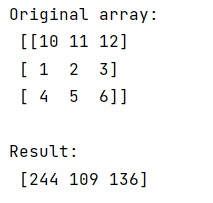# Iterating over NumPy matrix rows to apply a function each?

Learn, how to iterate over NumPy matrix rows to apply a function each in Python?
Submitted by Pranit Sharma, on January 25, 2023

NumPy is an abbreviated form of Numerical Python. It is used for different types of scientific operations in python. Numpy is a vast library in python which is used for almost every kind of scientific or mathematical operation. It is itself an array which is a collection of various methods and functions for processing the arrays.

## NumPy Matrix - Iterating over rows to apply a function each

Suppose that we are given a NumPy matrix, and, we have a self-defined function. We need to apply this function on each row of this matrix and hence we need to iterate over this matrix.

We can use numpy.apply_along_axis(). Assuming that our array is 2D, we can use this method which will automatically apply the function along the rows of this matrix.

Let us understand with the help of an example,

## Python code to iterate over NumPy matrix rows to apply a function each

```# Import numpy
import numpy as np

# Creating a numpy array
arr = np.array([[10, 11, 12],[1, 2, 3],[4, 5, 6]])

# Display original array
print("Original array:\n",arr,"\n")

# defining a function
def fun(x):
return x**2+100

# Applying function to each row
res = np.apply_along_axis(fun, axis=1, arr=arr)

# Display result
print("Result:\n",res)
```

Output: